### 5. INHOMOGENEITIES ON SUPERCLUSTER (AND LARGER) SCALES

On the larger scales of superclusters, we cannot assume that the systems are virialised, but the gravitational effect of the excess density will cause velocity perturbations which are in principle measurable. For a spherical perturbation where the overdensity is not large enough to cause turnaround, the velocity perturbation is related to the overdensity, and to, by the following equation.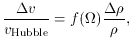(1)

where f()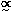0.6. The overdensity factor/may not be equal to the overdensity in luminous galaxies. Indeed it is customary to regard these quantities as related by a bias factor b. Our local peculiar velocity, measurable by our motion through the microwave background, is generally thought to have been induced by inhomogeneities in the distribution of galaxies around us. Clusters pull, and voids in effect 'push', our Local Group.

We can readily infer, since gravity and light both obey inverse square laws, that the contributions to our peculiar velocity made by any particular galaxy or group is proportional to the light which we receive from it, provided that b is indeed a constant. We note also that the gravitational potential fluctuation (M/R) required to cause a given dv (M/R2) is larger if the scale is larger.

The above equation can be applied to our own local motion just from two-dimensional data, without knowledge of the redshifts of galaxies. The next step, however, is a much more ambitious one, namely to apply the equation to infer the mass distribution throughout our local region of space, given adequate knowledge of the peculiar velocities of all the galaxies. The peculiar velocities can, of course, only be determined if one has a distance indicator which is sufficiently precise to distinguish between the actual distance of a galaxy and the distance it would have if it was obeying the Hubble flow. The main practical uncertainties in the measurement and interpretation of largescale streaming stem from the problems of calibrating the distance indicators.

In principle, however, knowledge of the largescale velocity field would allow us to infer the mass distribution without any assumption that galaxies trace mass, or that the quantity b is a universal ratio. We need only assume that galaxies are test particles whose motions are gravitationally induced. The main problems are still with the distance measurements, and a lingering uncertainty about whether the luminosity calibrators (velocity dispersion, diameter, etc.) are really independent of location and environment.

The expected deviations from Hubble flow are only a few hundred kilometers per second at most. There is very little hope of ever measuring these reliably by existing methods at distances beyond ~ 100 megaparsecs, because they are then only a very small fraction of the recession velocity, and therefore would require still more accurate distance indicators. There are, however, in principle other quite different methods which could determine peculiar velocities of clusters at still greater distances. For instance, there is an extra contribution to the Sunyaev-Zeldovich effect due to the bulk motion of the cluster, This is equivalent to a simple 'moving mirror' effect, with amplitude equal to the Doppler shift multiplied by the Compton optical depth. (Transverse motions, incidentally, would yield polarization, but this is a higher order effect and still more challenging for observers.)

What we would really like would be a method of directly delineating the dark matter distribution on large scales. One possibility which has recently been suggested is gravitational distortion of faint background galaxies. A supercluster would not have such a high column density as the core of a rich cluster, and therefore could not give strongly distorted or highly magnified images resembling the 'arcs'. But even if the induced distribution were small the fact that the angular diameter of a supercluster may be of order 1° means that up to 105 galaxies would share a correlated distortion; so it is by no means hopeless to look for an effect of only a few per cent.

At the moment one cannot conclusively decide between two extreme views regarding the dark matter distribution. On the one hand, it could be that on large scales the overall mass distribution is smooth, and the apparent largescale structure in the galaxy distribution represents simply some largescale spatial modulation of the efficiency of galaxy formation. On the other hand, the density of galaxies could be a reliable tracer of the dark matter distribution on all scales, except for a simple biasing factor b.

We can nevertheless say with great confidence that on scales much larger than 50 megaparsecs the overall mass distribution must be relatively smooth, in the sense that the amplitude of the r.m.s. density fluctuations is << 1. Our best evidence here comes from the microwave background anisotropies. For scales exceeding the horizon scale at the epoch of recombination, the dominant contribution toT/T comes from the gravitational potential or metric fluctuations on the last scattering surface. This is the so-called 'Sachs-Wolfe' effect. The upper limits are of order 10-5, and these imply that on the corresponding scale L(~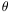ctH) we must have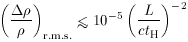(2)

Even a mass concentration as large as the Great Attractor would, if placed on the last scattering surface, yield a fluctuation little more than 10-5.

The microwave background anisotropies also, as (2) implies, constrain the potential fluctuation on mass much larger than 100 megaparsecs, This is of interest because it allows us to test whether the initial fluctuation spectrum does indeed have a power law form. The assumption of a power law is generally made because it seems unnatural to feed the mass scale appropriate to clusters or galaxies into the conditions of the early universe. Figure 5 summarizes the various constraints on the fluctuation amplitude on scales stretching all the way from sub-stellar masses up to the Hubble radius and beyond. We see that if the initial fluctuation spectrum is a power law, its slope is well constrained. Moreover, if it is a power law with the scale-independent Harrison-Zeldovich form, the amplitude is well constrained to be a bit less than 10-5. It is perhaps worth noting, however, that the case for favoring a Harrison-Zeldovich spectrum would be very much weaker if the universe were not, as theorists like to believe, flat with exactly the critical density. Ifdiffers significantly from unity, there is a preferred length scale in the universe, essentially the Robertson-Walker curvature radius. Given that this scale, a substantial fraction of the present Hubble radius, would have to have been imprinted at an early time, it would then be by no means implausible to suppose that other scales, related to it by some modest numerical factor, might also have been singled out.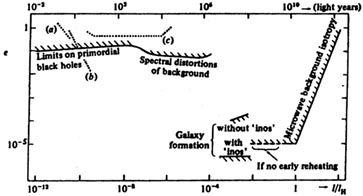Figure 5. This diagram depicts the astrophysical limits on the amplitudeof adiabatic metric fluctuations, on various scales l. On large scales, the microwave background isotropy offers stringent upper limits. On very small scales,must merely be not so large that too much of the universe collapses as the relevant scales enter the horizon; the absence of distortion in the microwave background sets a slightly better limit for mass-scales 104 - 1013 M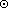. The requirement that bound systems have 'turned around' by the present epoch gives lower limits (also plotted). A spectrum with10-5 on all scales is acceptable if the universe is dominated by non-baryonic matter which can start clustering before trec. Ifhas a power-law dependence on l and is ~ 10-5 on the scales relevant to galaxy formation, then it cannot fall off more steeply thanl-0.15 without causing excessive production of primordial mini-holes. Also plotted (dotted lines) are the amplitudesg of primordial gravitational waves that can, within the next few years, be probed by: (a) doppler tracking of spacecraft, (b) timing of 'quiet' pulsars, and (c) timing of the orbit of the binary pulsar. This diagram is drawn assuming a 'flat' background universe with0 = 1. If0 < 1 the limits on large scales are modified. Indeed the appropriate definition ofis then somewhat ambiguous on scales exceeding the Robertson-Walker curvature radius.

It is interesting to ask whether a nearby cluster or supercluster would perturb the microwave background. The Sachs-Wolfe effect due to the precursor of a cluster on the last scattering surface causes a temperature perturbation of order/ c2, whereis the Newtonian potential; this is of order v2 / c2 where v is the velocity dispersion in the cluster after virialisation.

But a similar cluster with redshift z < 1 produces a much smaller effect on the microwave background. The effect of the cluster's potential well on the microwave photons is, to first order, zero: the photons gain energy falling in but lose it again climbing out the other side. The only non-zero effects are of higher order: they are due to the extra time delay experienced by photons going through the potential well of the cluster, and the deepening of the potential well as the photons go through. For a linear perturbation of radius r, these effects, the first a blueshift and the second a redshift, are both smaller than the Sachs-Wolfe effect by r / ctH. For a nonlinear collapsing perturbation the second effect may exceed the first by the ratio of the collapse rate to the Hubble expansion rate, but it is still generally much smaller than the Sachs-Wolfe effect that would be associated with a similar cluster observed in its embryonic state on the surface of last scattering.